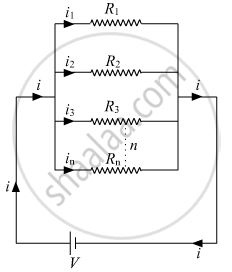# Answer the Following Question. with the Help of a Suitable Circuit Diagram Prove that the Reciprocal of the Equivalent Resistance of a Group of Resistances Joined in Parallel is Equal to the Sum of - Science

With the help of a suitable circuit diagram prove that the reciprocal of the equivalent resistance of a group of resistances joined in parallel is equal to the sum of the reciprocals of the individual resistances.

#### SolutionLet there are n resistances, each of value RI, R2 Rn, respectively, are connected in parallel to a battery of voltage V. if the equivalent resistance of the circuit is Req, then-current drawn from
"i" = "V"/"R"_("eq")
the battery is
The total current /then divides into Ii, i2, i3 in, respectively in the given resistors. As all the resistances are connected in parallel, hence the voltage across each resistor is V Volt. Now we can write,
i= i1 + i2 + i3 + ...........+ ieq

"V"/"R"_("eq")="V"/"R"_1+"V"/"R"_2+"V"/"R"_3.......+ "V"/"R"_"n"   ......(1)

From eq. 1,

1/"R"_("eq")=1/"R"_1+1/"R"_2+1/"R"_3......+1/"R"_"n"
Hence, reciprocal of the equivalent resistance is equal to the sum of reciprocal of each resistor joined in parallel

Concept: Electric Circuit
Is there an error in this question or solution?